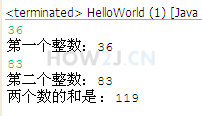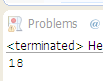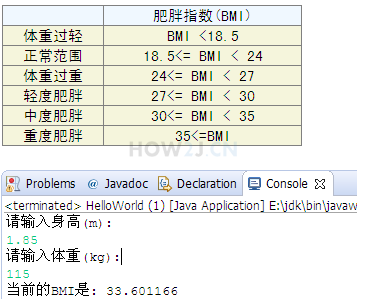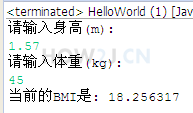+ - * / %

++ --13分21秒

## 基本算数操作符

+ - * /

public class HelloWorld { public static void main(String[] args) { int i = 10; int j = 5; int a = i+j; int b = i - j; int c = i*j; int d = i /j; } }
```public class HelloWorld {
public static void main(String[] args) {
int i = 10;
int j = 5;
int a = i+j;
int b = i - j;
int c = i*j;
int d = i /j;
}
}
```

## 练习-求和

姿势不对,事倍功半! 点击查看做练习的正确姿势## 答案-求和2分2秒 本视频采用html5方式播放，如无法正常播放，请将浏览器升级至最新版本，推荐火狐，chrome，360浏览器。 如果装有迅雷，播放视频呈现直接下载状态，请调整 迅雷系统设置-基本设置-启动-监视全部浏览器 (去掉这个选项)。 chrome 的 视频下载插件会影响播放，如 IDM 等，请关闭或者切换其他浏览器import java.util.Scanner; public class HelloWorld { public static void main(String[] args) { Scanner s = new Scanner(System.in); int a = s.nextInt(); System.out.println("第一个整数："+a); int b = s.nextInt(); System.out.println("第二个整数："+b); int c = a+b; System.out.println("两个数的和是 ：" + c); } }
```import java.util.Scanner;

public class HelloWorld {
public static void main(String[] args) {
Scanner s = new Scanner(System.in);
int a = s.nextInt();
System.out.println("第一个整数："+a);
int b = s.nextInt();
System.out.println("第二个整数："+b);
int c = a+b;
System.out.println("两个数的和是 ：" + c);
}
}
```

## 任意运算单元的长度超过int

int a = 5;
long b = 6;
a+b -> 结果类型是long
public class HelloWorld { public static void main(String[] args) { int a = 5; long b = 6; int c = (int) (a+b); //a+b的运算结果是long型，所以要进行强制转换 long d = a+b; } }
```public class HelloWorld {
public static void main(String[] args) {

int a = 5;
long b = 6;
int c = (int) (a+b); //a+b的运算结果是long型，所以要进行强制转换
long d = a+b;

}
}
```

## 任意运算单元的长度小于int

byte a = 1;
byte b= 2;
a+b -> int 类型
public class HelloWorld { public static void main(String[] args) { byte a = 1; byte b= 2; byte c = (byte) (a+b); //虽然a b都是byte类型，但是运算结果是int类型，需要进行强制转换 int d = a+b; } }
```public class HelloWorld {
public static void main(String[] args) {
byte a = 1;
byte b= 2;
byte c = (byte) (a+b); //虽然a b都是byte类型，但是运算结果是int类型，需要进行强制转换
int d = a+b;
}
}
```

## %取模

% 取余数，又叫取模
5除以2，余1
public class HelloWorld { public static void main(String[] args) { int i = 5; int j = 2; System.out.println(i%j); //输出为1 } }
```public class HelloWorld {
public static void main(String[] args) {

int i = 5;
int j = 2;
System.out.println(i%j); //输出为1
}
}
```

## 自增 自减

++
--

public class HelloWorld { public static void main(String[] args) { int i = 5; i++; System.out.println(i);//输出为6 } }
```public class HelloWorld {
public static void main(String[] args) {

int i = 5;
i++;
System.out.println(i);//输出为6

}
}
```

## 自增 自减操作符置前以及置后的区别

int i = 5;
i++; 先取值，再运算
++i; 先运算，再取值
public class HelloWorld { public static void main(String[] args) { int i = 5; System.out.println(i++); //输出5 System.out.println(i); //输出6 int j = 5; System.out.println(++j); //输出6 System.out.println(j); //输出6 } }
```public class HelloWorld {
public static void main(String[] args) {
int i = 5;
System.out.println(i++); //输出5
System.out.println(i);   //输出6

int j = 5;
System.out.println(++j); //输出6
System.out.println(j);	 //输出6
}
}
```

## 练习-自增

姿势不对,事倍功半! 点击查看做练习的正确姿势

int i = 1;
int j = ++i + i++ + ++i + ++i + i++;

## 答案-自增2分26秒 本视频采用html5方式播放，如无法正常播放，请将浏览器升级至最新版本，推荐火狐，chrome，360浏览器。 如果装有迅雷，播放视频呈现直接下载状态，请调整 迅雷系统设置-基本设置-启动-监视全部浏览器 (去掉这个选项)。 chrome 的 视频下载插件会影响播放，如 IDM 等，请关闭或者切换其他浏览器public class HelloWorld { public static void main(String[] args) { int i = 1; int j = ++i + i++ + ++i + ++i + i++; //i值 2 3 4 5 6 //取值 2 2 4 5 5 System.out.println(j); } }
```public class HelloWorld {
public static void main(String[] args) {
int i = 1;
int j = ++i + i++ + ++i + ++i + i++;
//i值        2     3     4     5     6
//取值      2     2     4     5     5
System.out.println(j);
}
}
```

## 练习-BMI

姿势不对,事倍功半! 点击查看做练习的正确姿势

BMI的计算公式是 体重(kg) / (身高*身高)

72 / (1.69*1.69) = ?## 答案-BMI4分19秒 本视频采用html5方式播放，如无法正常播放，请将浏览器升级至最新版本，推荐火狐，chrome，360浏览器。 如果装有迅雷，播放视频呈现直接下载状态，请调整 迅雷系统设置-基本设置-启动-监视全部浏览器 (去掉这个选项)。 chrome 的 视频下载插件会影响播放，如 IDM 等，请关闭或者切换其他浏览器import java.util.Scanner; public class HelloWorld { public static void main(String[] args) { Scanner s = new Scanner(System.in); System.out.println("请输入身高(m):"); float height = s.nextFloat(); System.out.println("请输入体重(kg):"); float weight = s.nextFloat(); float BMI = weight/ (height*height); System.out.println("当前的BMI是: " + BMI); } }
```import java.util.Scanner;

public class HelloWorld {
public static void main(String[] args) {
Scanner s = new Scanner(System.in);
System.out.println("请输入身高(m):");
float height = s.nextFloat();
System.out.println("请输入体重(kg):");
float weight = s.nextFloat();
float BMI = weight/ (height*height);
System.out.println("当前的BMI是: " + BMI);
}
}
```

HOW2J公众号，关注后实时获知最新的教程和优惠活动，谢谢。2022-07-19 请问大家为什么我的浮点数必须要用“,”代替“.”才能运算出结果呀？
 akashi12201018 我的浮点数只有输入“,"比如这样： Please enter your height: 1,85 Please enter your weight(kg): 115 Your BMI is: 33.601166 才能得出正常结果， 但如果我打“."，比如这样： Please enter your height: 1.85 Please enter your weight(kg): 115 Exception in thread "main" java.util.InputMismatchException at java.base/java.util.Scanner.throwFor(Scanner.java:939) at java.base/java.util.Scanner.next(Scanner.java:1594) at java.base/java.util.Scanner.nextFloat(Scanner.java:2496) at myfile.Testname.main(Testname.java:9) 就会报错 先谢谢大佬们解答啦！ ```package myfile; import java.util.Scanner; public class Testname { public static void main(String[] args) { Scanner s = new Scanner(System.in); System.out.println("Please enter your height(meter): "); float height = s.nextFloat(); System.out.println("Please enter your weight(kg): "); float weight= s.nextFloat(); System.out.println("Your BMI is: "); System.out.println(weight/(height*height)); s.close(); } } ``` ```Please enter your height: 1,85 Please enter your weight(kg): 115 Your BMI is: 33.601166```

2 个答案

1234567890xc

public static void main(String[] args) { System.out.println("请输入你的身高（m）："); System.out.println("亲输入你的体重（kg）："); Scanner v=new Scanner(System.in); float m=v.nextFloat(); System.out.println(m); float z=v.nextFloat(); System.out.println(z); final float BIM=z/(m*m); if (BIM<18.5) { System.out.println("当前的BIM"+BIM+"体重过轻"); } else if (18.5<=BIM&&BIM<24) { System.out.println("当前的BIM"+BIM+"正常范围"); }else if (24<=BIM&&BIM<27) { System.out.println("当前的BIM"+BIM+"体重过重"); }else if (24<=BIM&&BIM<27) { System.out.println("当前的BIM"+BIM+"轻度肥胖"); } else if (24<=BIM&&BIM<27) { System.out.println("当前的BIM"+BIM+"中度肥胖"); }else if (24<=BIM&&BIM<27) { System.out.println("当前的BIM"+BIM+"重度肥胖"); } System.out.println("你的体重是："+BIM); }

public class demo_BMI { public static void main(String[] args) { Scanner scn=new Scanner(System.in); System.out.println("请输入身高（m）:"); float sg=scn.nextFloat(); System.out.println("请输入体重（kg）:"); int tz=scn.nextInt(); final float BMI=tz/(sg*sg); if(BMI<18.8) { System.out.println("当前的BMI是："+BMI+"体重过轻"); }else if(BMI>=18.5 && BMI<24) { System.out.println("当前的BMI是："+BMI+"正常范围"); }else if(BMI>=24 && BMI <27) { System.out.println("当前的BMI是："+BMI+"体重过重"); }else if(BMI>=27 && BMI <30) { System.out.println("当前的BMI是："+BMI+"轻度肥胖"); }else if(BMI>=30 && BMI <35) { System.out.println("当前的BMI是："+BMI+"中度肥胖"); }else if(BMI>=35) { System.out.println("当前的BMI是："+BMI+"重度肥胖"); } } }
```请输入身高（m）:
1.72

90

2022-07-15 练习题输出结果不正确，输出了0是怎么回事呀
 juliewww 为什么输出Bmi值是0呀qwq ```import java.util.Scanner; public class BMI { public static void main(String[] args){ System.out.println("请输入身高（m）："); Scanner height = new Scanner(System.in); int l = height.nextInt(); System.out.println("请输入体重（kg）："); Scanner weight = new Scanner(System.in); int m = weight.nextInt(); float BMI = m / (l * l); System.out.println("当前BMI值是："+BMI); } }``` ```Description Resource Path Location Type Resource leak: 'weight' is never closed BMI.java /java/src line 10 Java Problem Description Resource Path Location Type Resource leak: 'height' is never closed BMI.java /java/src line 6 Java Problem ```

3 个答案

13782193172b

Scanner s = new Scanner(System.in); System.out.println("请输入身高"); float a=s.nextFloat(); System.out.println("请输入体重"); int b=s.nextInt(); final float Bmi=b/(a*a); if (Bmi<18.5){ System.out.println("当前的BMI是："+Bmi+"体重过轻"); }else if (Bmi<24&&Bmi>=18.5){ System.out.println("当前的BMI是："+Bmi+"正常范围"); }else if(Bmi>=24 && Bmi <27) { System.out.println("当前的BMI是："+Bmi+"体重过重"); }else if(Bmi>=27 && Bmi <30) { System.out.println("当前的BMI是："+Bmi+"轻度肥胖"); }else if(Bmi>=30 && Bmi <35) { System.out.println("当前的BMI是："+Bmi+"中度肥胖"); }else if(Bmi>=35) { System.out.println("当前的BMI是："+Bmi+"重度肥胖"); } }

jerrytoy114

2022-04-14 大家看看
2021-12-15 为什么赋值a不换行，而是a+'\n'进行运算得出20330
2021-11-21 优先级